# 0708-1300/Class notes for Tuesday, April 1

(diff) ← Older revision | Latest revision (diff) | Newer revision → (diff)
Announcements go here

## Typed Notes

The notes below are by the students and for the students. Hopefully they are useful, but they come with no guarantee of any kind.

### First Hour

Theorem

If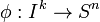$\phi:I^k\rightarrow S^n$ is an embbeding then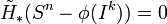$\tilde{H}_*(S^n-\phi(I^k)) = 0$

Proof:

By induction on k.

For k=0 this is easy,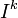$I^k$ is a single point and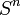$S^n$ - a point is just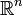$\mathbb{R}^n$

Now suppose we know the theorem is true for k-1, let$\phi:I^k\rightarrow S^n$ be an embedding. Write the cube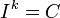$I^k = C$ as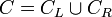$C=C_L\cup C_R$ where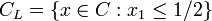$C_L=\{x\in C:x_1\leq 1/2\}$ and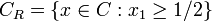$C_R=\{x\in C:x_1\geq 1/2\}$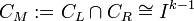$C_M:= C_L\cap C_R\cong I^{k-1}$

So,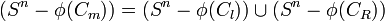$(S^n-\phi(C_m)) = (S^n-\phi(C_l))\cup(S^n-\phi(C_R))$

where both sets in the union are open. Recall for sets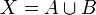$X = A\cup B$ where A and B are open we have the Mayer Vietoris Sequence.

Note: It is usefull to "open the black box" and think about the Mayer Vietoris Sequence from the veiwpoint of singular homology, ie using maps of simplexes and the like. This was briefly sketched in class.

We get a sequence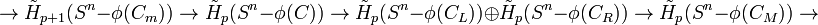$\rightarrow\tilde{H}_{p+1}(S^n-\phi(C_m))\rightarrow \tilde{H}_{p}(S^n-\phi(C))\rightarrow \tilde{H}_{p}(S^n-\phi(C_L))\oplus \tilde{H}_{p}(S^n-\phi(C_R))\rightarrow \tilde{H}_{p}(S^n-\phi(C_M))\rightarrow$

The first and last terms in this sequence (at least the small part of it we have written) vanish by induction hypothesis.

Note: Technically we need to check at Mayer Vietoris sequence also works for reduced homology.

We thus get the isomorphism: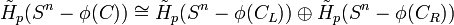$\tilde{H}_{p}(S^n-\phi(C))\cong \tilde{H}_{p}(S^n-\phi(C_L))\oplus \tilde{H}_{p}(S^n-\phi(C_R))$

Assume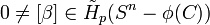$0\neq[\beta]\in \tilde{H}_{p}(S^n-\phi(C))$ As the above isormorphism is induced by the inclusion maps at the chain level, we get that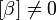$[\beta]\neq 0$ either in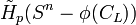$\tilde{H}_{p}(S^n-\phi(C_L))$ or in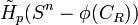$\tilde{H}_{p}(S^n-\phi(C_R))$

Now repeat, find a sequence of intervals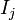$I_j$ such that

1)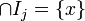$\cap I_j = \{x\}$

2)$[\beta]\neq 0$ in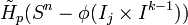$\tilde{H}_{p}(S^n-\phi(I_j\times I^{k-1}))$

Pictorially, we cut C in half, find which half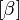$[\beta]$ is non zero, then cut this half in half and find the one the$[\beta]$ is non zero in and again cut it in half, etc...

But,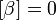$[\beta] = 0$ in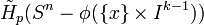$\tilde{H}_{p}(S^n-\phi(\{x\}\times I^{k-1}))$ by induction. Hence,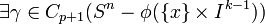$\exists\gamma\in C_{p+1}(S^n-\phi(\{x\}\times I^{k-1}))$ such that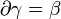$\partial\gamma=\beta$.

But,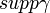$supp\gamma$ = { union of images of simplexes in U} is compact, so,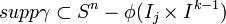$supp\gamma\subset S^n-\phi(I_j\times I^{k-1})$ for some j. But this contradicts assumption 2)

Q.E.D

We now prove an analogous theorem for spheres opposed to cubes

Theorem

For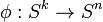$\phi:S^k\rightarrow S^n$ an embedding then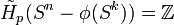$\tilde{H}_{p}(S^n-\phi(S^k)) = \mathbb{Z}$ if p = n-k-1 and zero otherwise.

Intuitively,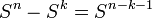$S^n-S^k = S^{n-k-1}$

Recall we had seen this explicitly for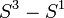$S^3-S^1$ when we wrote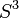$S^3$ as the union of two tori (an inflated circle)

Proof:

As in the previous proof, we use Mayer Vietoris. Let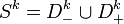$S^k = D^k_-\cup D^k_+$

Then,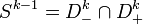$S^{k-1} = D^k_-\cap D^k_+$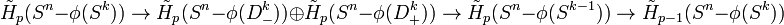$\tilde{H}_{p}(S^n-\phi(S^k))\rightarrow \tilde{H}_{p}(S^n-\phi(D^k_-))\oplus\tilde{H}_{p}(S^n-\phi(D^k_+))\rightarrow \tilde{H}_{p}(S^n-\phi(S^{k-1}))\rightarrow \tilde{H}_{p-1}(S^n-\phi(S^k))$

The first and last term written vanish by induction hypothesis and so have an isomorphism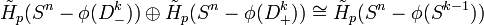$\tilde{H}_{p}(S^n-\phi(D^k_-))\oplus\tilde{H}_{p}(S^n-\phi(D^k_+))\cong \tilde{H}_{p}(S^n-\phi(S^{k-1}))$

Hence, it is enough to prove the theorem for k=0. Well in this case we have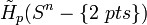$\tilde{H}_{p}(S^n-\{2\ pts\})$

Since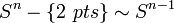$S^n-\{2\ pts\}\sim S^{n-1}$, this is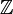$\mathbb{Z}$ for p = n-1 and 0 otherwise

Q.E.D

Take k=n-1$\tilde{H}_{0}(S^n-\phi(S^{n-1})) =\mathbb{Z}$ so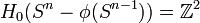$H_{0}(S^n-\phi(S^{n-1}))=\mathbb{Z}^2$

Hence the compliment of an n-1 sphere in$S^n$ has two connected components. This is the Jordan Curve Theorem.